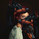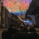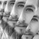צפיות ‎2233‎
This study is an experiment in adaptive filtering. The process in this study was inspired by KAMA and ZLEMA filtering techniques.
First, data is given an optional modification for lag reduction.
Then, an adaptive filter of your choice is calculated. There are 6 different adaptive filters to choose from in this study:
-Commodity Channel Index Adaptive Moving Average (CCIAMA)
-Relative Strength Index Adaptive Moving Average (RSIAMA)
-Klinger Volume Oscillator Adaptive Moving Average (KVOAMA)
-Money Flow Index Adaptive Moving Average (MFIAMA)
-Correlation Coefficient Adaptive Moving Average (CCAMA)
Next, ATR is calculated using the specified adaptive filter.
A set of ranges is calculated by multiplying ATR by the square root of the sampling period, then dividing it by 2 and 4.
And Finally, the ranges are added to and subtracted from the adaptive filter to generate the channels.

Custom bar colors are included. The formula for the color scheme is based on filter direction and price.

After analyzing the original code more in depth, I realized a few flaws with the method I previously used, so I decided to rebuild the whole thing from scratch.
This version is significantly more versatile and adaptive to data.

In this version, rather than using altered index data to operate the smoothing parameters, I've applied Kaufman's Efficiency Ratio to a handful of indices.
The smoothing operators available in this script are:
- Kaufman's Efficiency Ratio
- Time Correlation Efficiency Ratio
- Commodity Channel Index Efficiency Ratio
- Relative Strength Index Efficiency Ratio
- Klinger Volume Oscillator Efficiency Ratio
- Money Flow Index Efficiency Ratio
- Fisher Transform Efficiency Ratio
- Average True Range Efficiency Ratio
- Standard Deviation Efficiency Ratio

I also added a Variable Moving Average option per user request, which dynamically changes its smoothing period based on the operator.
Now you can choose between either an Adaptive Moving Average or Variable Moving Average as the calculation method.

The high and low bands are generated by calculating ATR based on the averaging method, multiplying it by a user defined coefficient, then adding and subtracting it from the basis average.
The smaller set of bands is generated by performing the same calculation with 78.6% ATR.

The bar color scheme is still based on filter direction and price.

I noticed the Variable Moving Average formula I used previously was an expanding model with a rather significant amount of overshoot, so I reverted to Tushar Chande's model.

I removed ATR Efficiency from the list, as it is not nearly as effective for this type of calculation as the other indices.

I've implemented some new methods for transforming operator data for different results:
- Standard, which uses the default Efficiency Ratio calculations
- Max Min Normalize, which normalizes the operator data based on maximum and minum values, resulting in a more sensitive response
- Inverse Fisher Normalize, which performs an Inverse Fisher Transform to the operator, then performs Max Min Normalization
- Gate, which changes all values below the gate to 0, resulting in a less sensitive response

The secondary band percentage for the high and low zones is now adjustable. The default value is now 61.8%.

The bar color scheme has been adjusted to highlight overbought and oversold conditions.
הערות שחרור: Not a big update. Just some simple housekeeping.

-> Migrated script to v4.
-> Reorganized script structure.
-> Smoothing operator calculations are now expressed as functions.
-> Added compression to the available smoothing operator modifications. Compression can be tuned by adjusting the threshold and intensity.
-> When using KVOER or MFIER, volume will be automatically substituted with tick volume when volume is NaN.
-> Corrected NaN values in initial states.
-> Revamped color scheme.

## תגובותI've just discovered you, you have a different approach. I congratulate you and respect you.
השבNice script! Would be cool if the period could also be adaptively tuned
השבmcbw_
@mcbw_, That does sound like a great idea, actually. I'll likely implement an update with that feature in the near future.
השבDonovanWall
@DonovanWall, That would be great, please let me know if you do!
השבmcbw_
@mcbw_, I did a complete overhaul on the script and included that update. You can now adaptively tune the period using the "VMA" option.
השבmcbw_
@mcbw_, If you checked out the previous version, the VMA algorithm was an expanding model. After closer inspection, I noticed there was a significant amount of overshoot in the calculation, so I reverted to Tushar Chande's model. The results are much better now.
השב